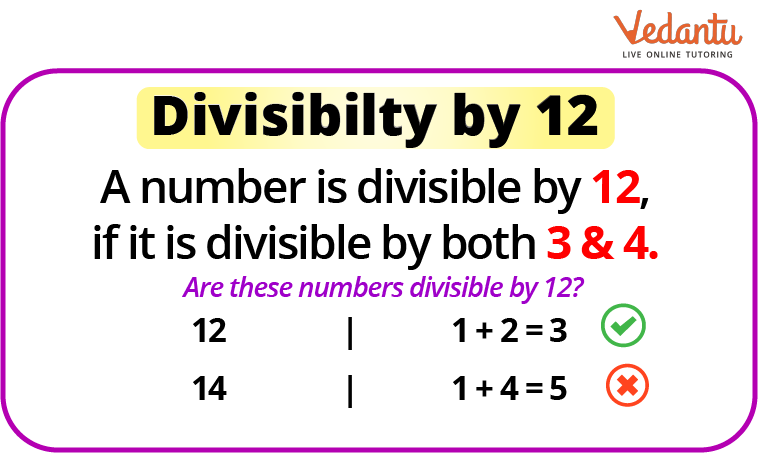Courses
Courses for Kids
Free study material
Free LIVE classes
More

# Numbers Divisible By 12LIVE
Join Vedantu’s FREE Mastercalss

## Introduction to Numbers

Imagine 3 friends trying to share 10 biscuits. Each of them gets 3 biscuits, and there’s one biscuit left over. They are unsure what to do with it; would one person get an extra biscuit? That did not seem fair to everyone, who loved sharing everything equally.

If there were 9 biscuits, they would have divided the biscuits equally, and there would have been no confusion. Because 9 is divisible by 3. This means that 9 biscuits could have been divided into three equal parts without any extra biscuits left.

## What is Divisibility Rule?

Divisibility rules are a set of general rules often used to determine whether or not a number is divisible by a particular number. Divisibility rules can help kids determine whether a number will be divisible by another.

## Divisibility Rule of 12

The divisibility chart or rule of 12 is -

If the number is divisible by both 3 and 4, then the number is divisible by 12 exactly.

Example: 5844

Sum of the digits = 5 + 8 + 4 + 4 = 21 (multiple of 3)

Last two digits = 44 (divisible by 4)

The number 5844 is divisible by 4 and 3; hence, it is divisible by 12.Divisibility by 12

## Solved Worksheet

Following are the divisibility rule of 12 with examples -

Example 1: Check whether 840 is divisible by 12 or not.

Solution: We know that -

If the given number is divisible by both 3 and 4, then it is also divisible by 12.

1. First, check whether the given number is divisible by 3.

Sum of the digits :8 + 4 + 0 = 12 (multiple of 3)

2. Now, check whether the given number is divisible by 4.

In the given number 840, the number formed by the last two digits is 40, divisible by 4. So, the number 840 is divisible by 4.

Now, it is clear that the given number 840 is divisible by both 3 and 4.

Therefore, the number 840 is divisible by 12

Example 2: Check whether 9140 is divisible by 12 or not.

Solution: We know that -

If the given number is divisible by both 3 and 4, then it is also divisible by 12.

1. Firstly, check whether the given number is divisible by 3.

Sum of the digits : 9 + 1 + 4 + 0 = 14 (not a multiple of 3)

Because the number 9140 is not divisible by 3, the number 9140 is not divisible by 12.

Example 3: Check whether 2370 is divisible by 12 or not.

Solution: We know that -

If the given number is divisible by both 3 & 4, then it is also divisible by 12.

1. divisible by 12.

1. Firstly, check whether the given number is divisible by 3.

Sum of the digits: 2 + 3 + 7 + 0 = 12 (multiple of 3)

2. Now, check whether the given number is divisible by 4.

In the given number 2370, the number formed by the last two digits is 70, which is not divisible by 4.

So, the number 2370 is not divisible by 4.

Therefore, the number 2370 is not divisible by 12.

## Conclusion

In this article, we have learned about the divisibility rule for 12. For instance, a number divisible by 12 will also be divisible by 3 and 4. Many mathematical rules and properties are required or beneficial to understand when solving a Maths problem. Learning and comprehending these rules provides students with a foundation to solve problems and tackle more advanced mathematical concepts.

Last updated date: 27th Sep 2023
Total views: 78.9k
Views today: 1.78k

## FAQs on Numbers Divisible By 12

1. What does the term "divisibility test" mean?

A divisibility test determines whether a given integer is divisible by a fixed divisor without performing the division, typically by examining its digits. A number is said to be divisible by another number if the result of the division is a whole number.

2. How many divisibility rules exist?

A divisibility rule is a heuristic for determining whether a positive integer can be divided evenly by another positive integer (i.e. there is no remainder left over). For example, determining whether a number is even is as simple as looking at its last digit: 2, 4, 6, 8, or 0.

3. What is the definition of a perfectly divisible number?

This method generally employs digits to determine whether a given number is divided by a divisor. If one number is perfectly divisible by another, the remainder should be zero, and the quotient should be a whole number. We have rules for divisibility for 1, 2, 3, 4, 5, 6, 7, 8, 9, 10, 11, 12, 13, and so on.